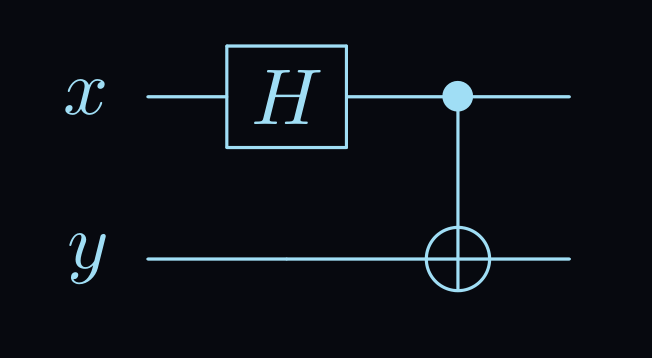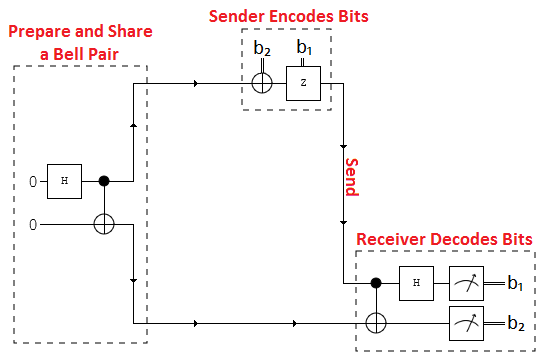in

# What is it?

Superdense Coding is a quantum communication protocol that allows for a sender to send two classical bits of information to another user by utilizing only one qubit. This algorithm takes advantage of something called a ‘bell circuit’. A bell circuit is a combination of a Hadamard gate going into the control bit of the controlled not gate; see image belowBell Circuit

A two qubit bell circuit will have an equal probability of getting either 00 or 11 as results. (Assuming the computer is noiseless.)

# How it works

In the superdense coding algorithm we have three different users.

User 1User 2User 3
The first user shares the two outputs of a two qubit bell state with the receiver and the sender.The second user who is the sender, encodes the qubits The final user receives those qubits and decodes them

With those rules in consideration, your final design will look like this;Superdense Coding Diagram - credit

A good thing to try and think about is how a bell state truly works. Think about it, the control bit is entangled with the not gate. So when we set the control bit into a super position it also changes the target bit to a superposition as well, because they are entangled.

We can denote the bell state mathematically like so

$\tfrac{1}{\sqrt{2}}(|00\rangle + |11\rangle)$

# The Encoder

The encoders job is simple, take the first qubit from the bell state and apply a single gate operation to it. In the two qubit example of this algorithm there are four different ways for the encoder to encode their qubit. Either with a Identity gate (does no change), X-gate, Z-gate, or a combination of the Z and X-gate. Each of these four different combinations represents an intended message.

MessageApplied GateResulting State
$$00$$$$I$$$$|00\rangle + |11\rangle$$
$$10$$$$X$$$$|01\rangle + |10\rangle$$
$$01$$$$Z$$$$|00\rangle - |11\rangle$$
$$11$$$$ZX$$$$-|01\rangle + |10\rangle$$

So if I the sender wanted to pass on the message of 10 to my receiver, I would apply an X-gate.

The receivers job is bit easier. All they have to do is take the untouched second qubit from the initial bell state and the new encoded qubit from the sender and pass it through a ‘reverse’ bell circuit.

the result will end up being what ever the sender has set their gate to. So in our example if the sender applied an X gate the receiver would decode the message and receive 10 as a result.

# Qiskit

Now that we have a foundation for what the superdense coding algorithm is, we can test it on a quantum computer simulator using Qiskit! If you do not have Qiskit installed yet, check out their official tutorial here.

### Printing the circuit

If you did everything correctly your output should look like the following;Superdense Coding Circuit Output

• The far left side of the circuit is your bell-state preparation
• The middle half with the ‘X’ gate is the Encoder
• And the to the far right you have your reverse bell-state, which is the receiver.
• And of course the measurement gates at the end to read the results.